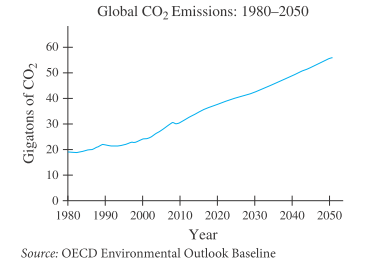# Fossil-fuel emissions Carbon in the atmosphere is due to carbon dioxide ( CO 2 ) emissions from fossil-fuel burning and is considered to be a primary contributor to climate change. Using data from the Organization for Economic Cooperation and Development (OECD) for selected years from 1980 and projected to 2050, global CO 2 emissions can be modeled by the differential equation d E d t = 0.0164 E where t is the number of years past 1980 and E is global CO 2 emissions (in gigatons). Solve this differential equation and find a particular solution that satisfies E ( 0 ) = 18.5 . Graph your solution and compare it with the following graph that shows OECD’s data and projections.### Mathematical Applications for the ...

11th Edition
Ronald J. Harshbarger + 1 other
Publisher: Cengage Learning
ISBN: 9781305108042

#### Solutions

Chapter
Section### Mathematical Applications for the ...

11th Edition
Ronald J. Harshbarger + 1 other
Publisher: Cengage Learning
ISBN: 9781305108042
Chapter 12.5, Problem 59E
Textbook Problem
1 views

## Fossil-fuel emissions Carbon in the atmosphere is due to carbon dioxide ( CO 2 ) emissions from fossil-fuel burning and is considered to be a primary contributor to climate change. Using data from the Organization for Economic Cooperation and Development (OECD) for selected years from 1980 and projected to 2050, global CO 2 emissions can be modeled by the differential equation d E d t = 0.0164 E where t is the number of years past 1980 and E is global CO 2 emissions (in gigatons). Solve this differential equation and find a particular solution that satisfies E ( 0 ) = 18.5 . Graph your solution and compare it with the following graph that shows OECD’s data and projections.This textbook solution is under construction.

### Still sussing out bartleby?

Check out a sample textbook solution.

See a sample solution

#### The Solution to Your Study Problems

Bartleby provides explanations to thousands of textbook problems written by our experts, many with advanced degrees!

Get Started